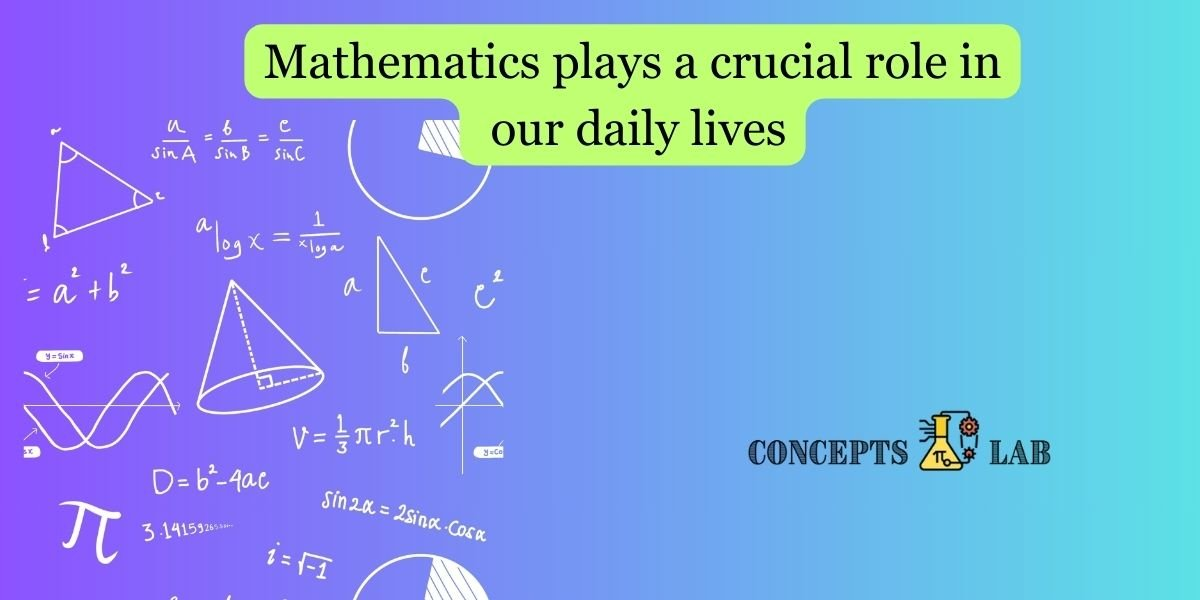Here are some common scenarios where mathematics is applied in daily life:

1. Budgeting:
• Managing personal finances involves budgeting, where you need to calculate income, expenses, savings, and make decisions based on these calculations.
2. Shopping:
• Calculating discounts, comparing prices, and determining the best value for money involve basic arithmetic skills.
3. Cooking:
• Measuring ingredients, adjusting recipes, and understanding cooking times all require mathematical skills.
4. Time Management:
• Planning daily schedules, setting alarms, and managing time effectively involve concepts of time and duration.
5. Travel:
• Calculating distances, estimating travel time, and understanding currency exchange rates are common mathematical tasks when planning a trip.
6. Home Improvement:
• Measuring spaces, calculating areas for painting or flooring, and budgeting for home improvement projects involve various mathematical concepts.
7. Health and Fitness:
• Monitoring calories, calculating body mass index (BMI), and tracking exercise routines involve mathematical calculations.
8. Technology Use:
• Understanding data usage, managing storage space, and troubleshooting technical issues often require mathematical skills.
9. Gardening:
• Planning garden layouts, calculating fertilizer quantities, and understanding growth patterns involve mathematical concepts.
10. Sports and Hobbies:
• Tracking scores, analyzing statistics, and understanding game strategies in sports involve mathematical reasoning.
11. Communication:
• Understanding time zones, calculating data usage for phone plans, and managing contacts all involve mathematical concepts.
12. Home Appliances:
• Setting temperatures, programming washing machines, and using household appliances often require numerical input and understanding.
13. Construction and DIY Projects:
• Measuring materials, calculating dimensions, and understanding angles are essential in construction and DIY projects.
14. Job and Career:
• Many professions, such as engineering, finance, science, and technology, heavily rely on mathematics.
15. Problem Solving:
• Math teaches problem-solving skills that are applicable in various aspects of life, from navigating challenges at work to resolving everyday issues.

By recognizing the importance of mathematics in daily life, individuals can develop a greater appreciation for the subject and its practical applications. Mathematics is a tool that empowers us to make informed decisions, solve problems, and navigate the complexities of the world around us.